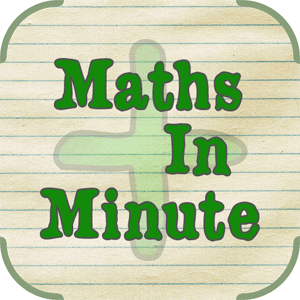# Maths in a minuteMaths in a minute is a mathematical game. In this game your mathematical skill will be tested.

How fast your mathematical calculations are? Here in this game, you will be bounded by a fixed time, within a minute there will be different mathematical calculations with corresponding answers shown.

Your goal is to click the correct one, your score will depend on how many true answers you are giving in a minute.